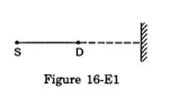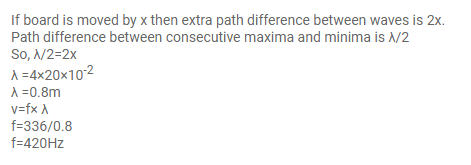# A source of sound S and detector D are placedQuestion:

A source of sound $S$ and detector $D$ are placed at some distance from one another. a big cardboard is placed near the detector and perpendicular to the line $S D$ as shown in figure. It is gradually moved away and it is found that the intensity changes from a maximum to a minimum as the board is moved through a distance of $20 \mathrm{~cm}$. Find the frequency of the sound emitted. Velocity of sound in air is $336 \mathrm{~m} \mathrm{~s}^{-1}$.Solution: